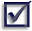If a temporary variable spans more than two or three lines, consider if it should be given something more specific than 'temp'.  eg tempTotal or total.

For example:

``` public Amount calculateTotalIncludingTax() throws Exception {     Amount temp = new Amount();     temp.add(sum);     temp.add(tax);     if (temp.isZero())     {         // this year is same as last year increased by default %         temp.setValue(lastTotal);         temp.multiply(YEARLY_MULTIPLIER);     }     return temp; } ```

If the temp variable was changed to give more detail, readability is enhanced:

``` public Amount calculateTotalIncludingTax() throws Exception {     Amount total = new Amount();     total.add(sum);     total.add(tax);     if (total.isZero())     {         // this year is same as last year increased by default %         total.setValue(lastTotal);         total.multiply(YEARLY_MULTIPLIER);     }     return total; } ```Tip: Note the use of the variable called 'total' instead of a more descriptive name.  The method is small, simple and well named so there is no chance the developer will be confused about what kind of total it is.